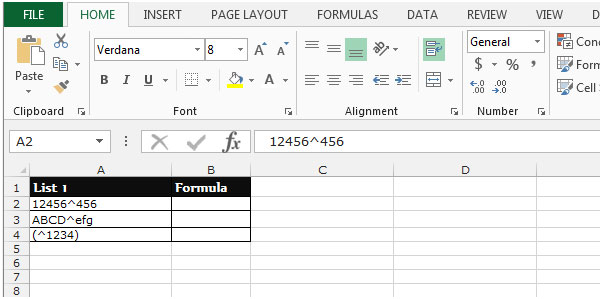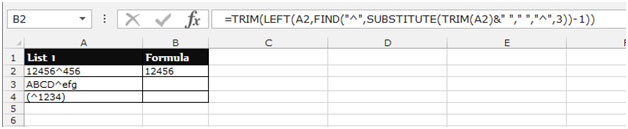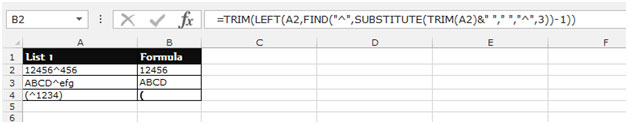# Extracting the First N Number of Words from a String in Excel

This article is about how to extract the first N number of words from a string in Microsoft Excel by using formulas. To solve this problem we will use TRIM, LEFT, FIND and SUBSTITUTE functions.

Let's see why we will use all of above mentioned formulas:-

FIND function helps to return the placed number at which a specific character or text string is first found.

LEFT function return the specified character from the defined cells from the left side of the string.

TRIM function is used to remove extra spaces from the cell or range.

SUBSTITUTE function is used to replace the old text with new text.

Let’s take an example to understand how we can extract all text before the tilde symbol (^).

This symbol appears at different locations in each string.

Example 1: We have a list in column A. We want to extract all text before the tilde (^) symbol whether it is text, number or symbols.  In this case, the number of characters we have to extract for each row vary.  It’s the same as extracting “N” number of words from a string, as the “N” can vary from string to string.• Select the Cell B2, write the formula.
• =TRIM(LEFT(A2,FIND("^",SUBSTITUTE(TRIM(A2)&" "," ","^",3))-1))
• Press Enter on your keyboard.
• The function will return the first N number.• To Copy the formula in all cells, press the key  “CTRL + C”  and select the cell B3:B4 and press the key “CTRL + V” on your keyboard.This is how we can extract a variable number of characters from a string in Microsoft Excel.